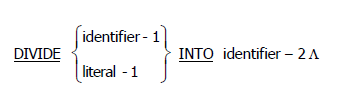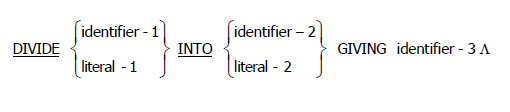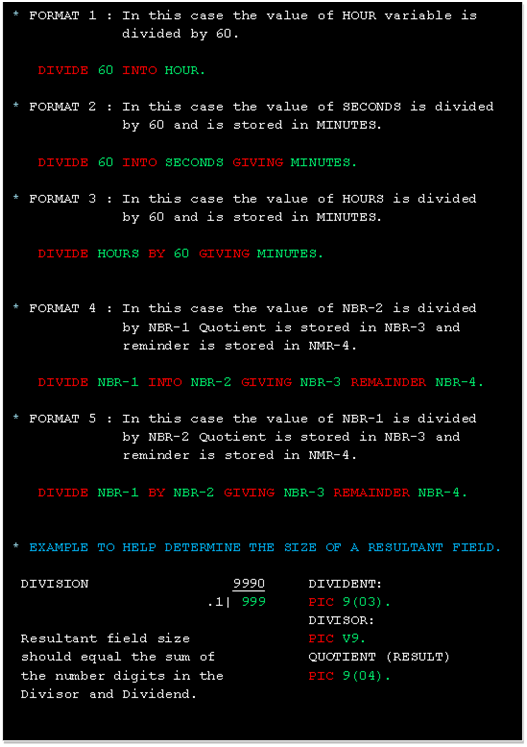## Thursday, 4 September 2014

### COBOL Divide Clause | COBOL Division Example.COBOL Divide Tutorial

In this tutorial, I would discussed another important COBOL verb i.e. DIVIDE cause, most you are already aware about.

The DIVIDE statement divides one numeric data item into or by other(s) and sets the values of data items equal to the quotient and remainder.

Divide clause  has five different format. Refer below figure for more details:

Format : 1COBOL Divide Clause

Here, the value of identifier-1 or literal-1 is divided into the value of identifier-2 and the quotient is then stored in identifier-2. For each successive occurrence of identifier-2, the division takes place in the left-to-right order in which identifier-2 is specified.

Format : 2COBOL Divide Clause

Here, the value of identifier-1 or literal-1 is divided into the value of identifier-2 or literal-2. The value of the quotient is stored in each data item referenced by identifier-3.

Format : 3COBOL Divide Clause

Here, the value of identifier-2 or literal-2 is divided into the value of identifier-1 or literal-1. The value of the quotient is stored in each data item referenced by identifier-3.

Format : 4COBOL Divide Clause

Here, the value of identifier-1 or literal-1 is divided into the value of identifier-2 or literal-2. The value of the quotient is stored in identifier-3 and the value of the remainder is stored in identifier-4.

Format : 5COBOL Divide Clause

Here, the value of identifier-1 or literal-1 is divided by the value of identifier-2 or literal-2. The value of the quotient is stored in identifier-3 and the value of the remainder is stored in identifier-4.

For more details refer below example:COBOL Divide Clause Example